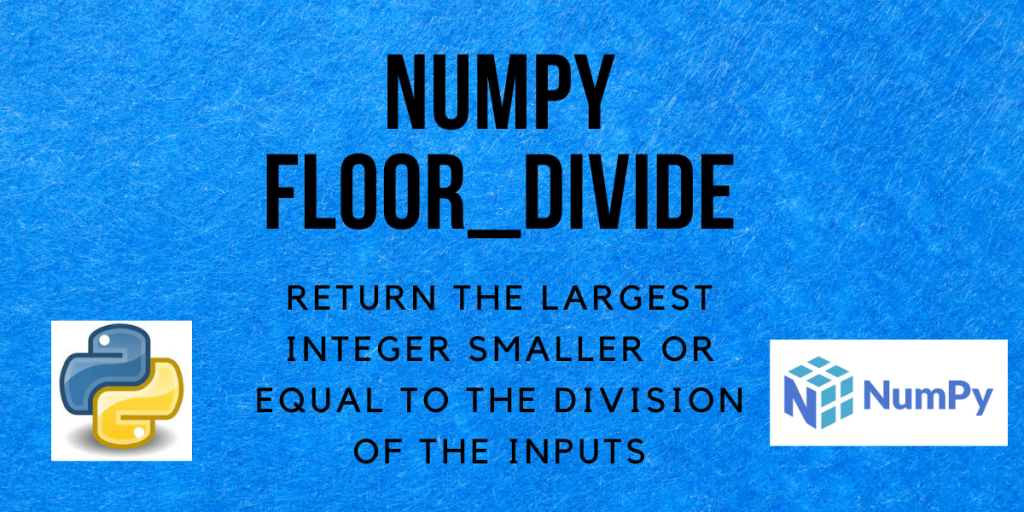# NumPy floor_divide – A Complete GuideHello and welcome to this tutorial on Numpy floor_divide. In this tutorial, we will be learning about the NumPy floor_divide() method and also seeing a lot of examples regarding the same. So let us begin!

## What is NumPy floor_divide?

The floor operator in Python is denoted by `//`. The NumPy `floor_divide` operator is a combination of `//` and `%` , then later begin the remainder or mod operator. The simplified equation is `a = a%b + b*(a//b)`.

The NumPy `floor_divide()` method returns the largest integer smaller than or equal to the division of the inputs. The inputs can be both arrays, or an array and a scalar.

We will see the examples for each of these in the upcoming section of this tutorial.

## Syntax of NumPy floor_divide

```numpy.floor_divide(x1, x2, out=None)
```

Returns:
An n-dimensional array y, `y=(x1/x2)`. y is a scalar if both x1 and x2 are scalars.

## Examples of NumPy floor_divide

Let’s now look at a few examples to understand the function better.

### When the divisor is a scalar

1-dimensional array

```import numpy as np

a = 5
arr = [12, 15, 10, 6]
ans = np.floor_divide(arr, a)
print("a =", a)
print("Result =", ans)
```

Output:

```a = 5
Result = [2 3 2 1]
```

The `floor_divide` method computes the output by dividing each element in the list by a and calculating its floor value of it. Here, 12/5 = 2.4, floor(2.4) = 2; 15/5 = 3, floor(3) = 3; 10/5 = 2, floor(2) = 2 and 6/5 = 1.2, floor(1.2) = 1.
Hence, the resulting array is [2, 3, 2, 1].

The // operator can be used as a shorthand for numpy.floor_divide on ndarrays.

```import numpy as np

a = 5
arr = np.array([12, 15, 10, 6])
ans = arr // a
print("a =", a)
print("Result =", ans)
```

Output:

```a = 5
Result = [2 3 2 1]
```

2-dimensional array

```import numpy as np

a = 5
arr = [[12, 15], [0, 36]]
ans = np.floor_divide(arr, a)
print("a =", a)
print("Result =\n", ans)
```

Output:

```a = 5
Result =
[[2 3]
[0 7]]
```

Similar to 1-dimensional arrays, here as well each element is divided by a and then its floor value is computed, which is stored in the result array.

• Result = floor(12/5) = floor(2.4) = 2
• Result = floor(15/5) = floor(3) = 3
• Result = floor(0/5) = floor(0) = 0
• Result = floor(36/5) = floor(7.2) = 7

### Division of 2 arrays/lists

```import numpy as np

arr1 = [10, 20, 30, 40]
arr2 = [4, 3, 6, 5]
ans = np.floor_divide(arr1, arr2)
print("Array 1 =", arr1)
print("Array 2 =", arr2)
print("Result =", ans)
```

Output:

```Array 1 = [10, 20, 30, 40]
Array 2 = [4, 3, 6, 5]
Result = [2 6 5 8]
```

In this case, the division and floor operation is performed on the corresponding elements in both arrays.

• Result =arr1/arr2 = floor(10/4) = floor(2.5) = 2
• Result =arr1/arr2 = floor(20/3) = floor(6.666) = 6
• Result =arr1/arr2 = floor(30/6) = floor(5) = 5
• Result =arr1/arr2 = floor(40/5) = floor(8) = 8

### When the arrays contain negative elements

Till now, we have seen examples in which all the elements were positive numbers. Now let us see how the `numpy.floor_divide()` method handles negative values.

```import numpy as np

arr1 = [16, 5, -30, 18]
arr2 = [8, -5, 7, 10]
ans = np.floor_divide(arr1, arr2)
print("Array 1 =", arr1)
print("Array 2 =", arr2)
print("Result =", ans)
```

Output:

```Array 1 = [16, 5, -30, 18]
Array 2 = [8, -5, 7, 10]
Result = [ 2 -1 -5  1]
```
• Result =arr1/arr2 = floor(16/8) = floor(2) = 2
• Result =arr1/arr2 = floor(5/-5) = floor(-1) = -1
• Result =arr1/arr2 = floor(-30/7) = floor(-4.2857) = -5
• Result =arr1/arr2 = floor(18/10) = floor(1.8) = 1

## Conclusion

That’s all! In this tutorial, we learned about the Numpy floor_divide method and practiced different types of examples using the same. If you want to learn more about NumPy, feel free to go through our NumPy tutorials.